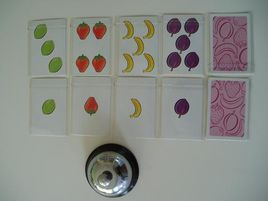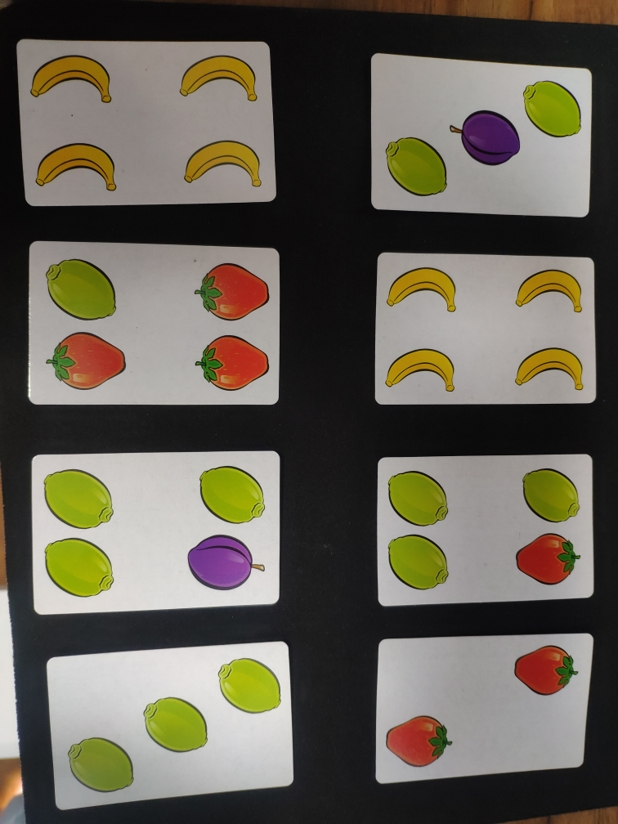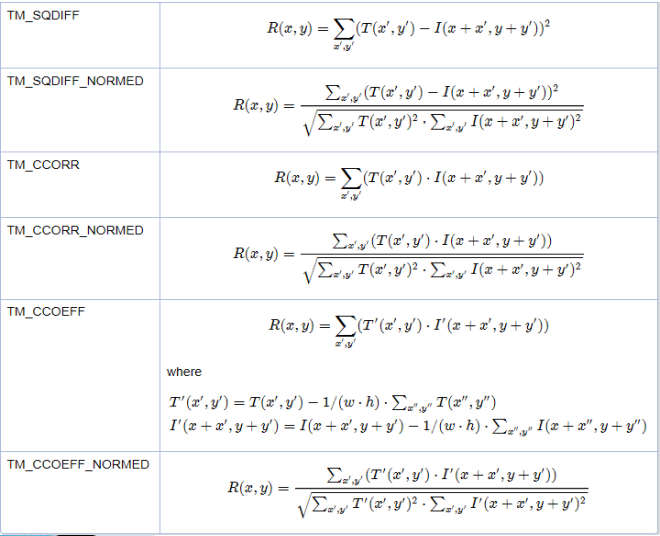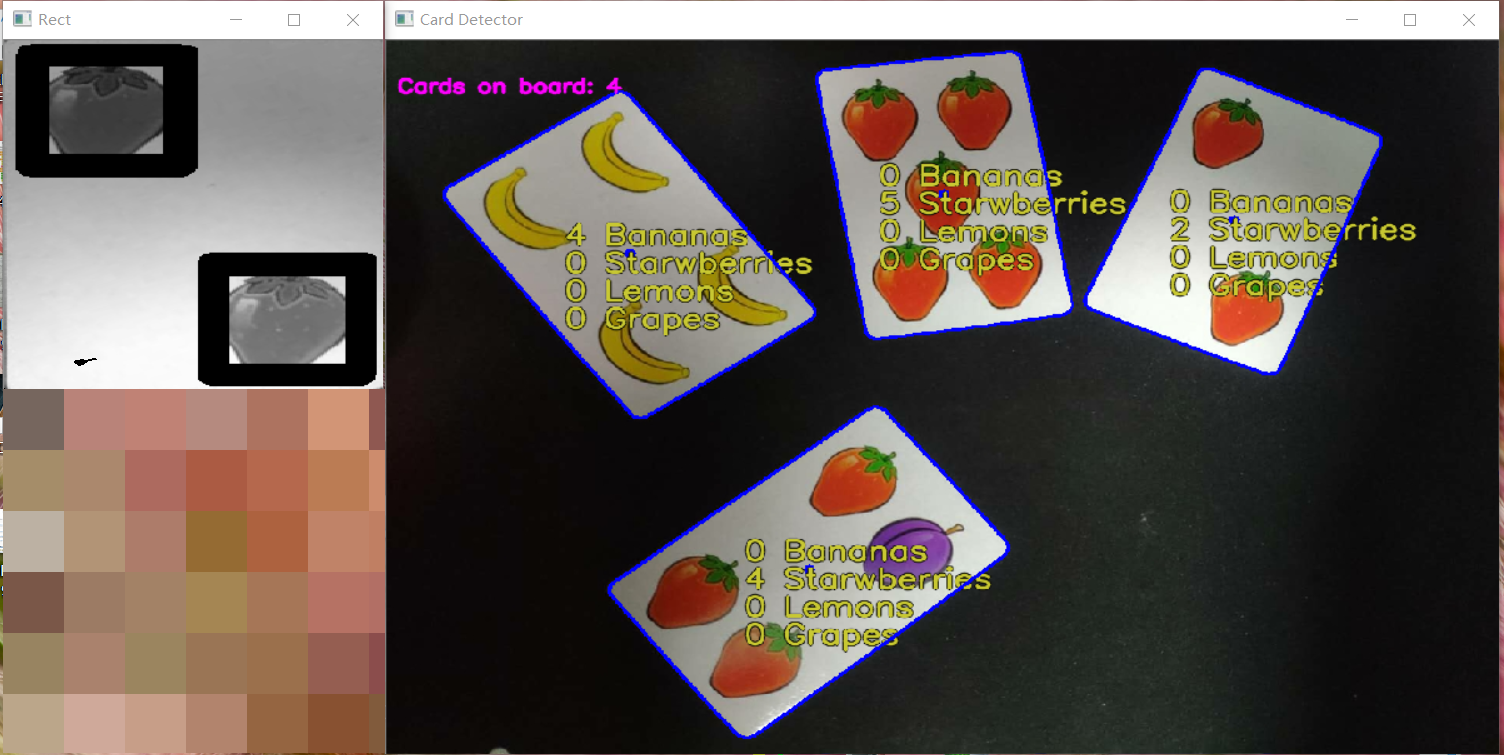## 引言## 游戏规则

1. 由于同一个玩家打出的卡牌在桌面上有遮挡关系，所以被遮挡的卡牌上的水果不计入总数。
2. 以上只是最基础的玩法，目前市面上的牌组加入了稀奇古怪的道具牌，不在本文及本项目的讨论范围。

## OpenCV思路cv2.findContours(image, mode, method[, contours[, hierarchy[, offset ]]])

• image-传入的二值图，请提前转成灰度再转为二值图
• mode-可选宏变量：
cv2.RETR_EXTERNAL 表示只检测外轮廓

cv2.RETR_LIST 检测的轮廓不建立等级关系
cv2.RETR_CCOMP 建立两个等级的轮廓，上面的一层为外边界，里面的一层为内孔的边界信息。如果内孔内还有一个连通物体，这个物体的边界也在顶层。
cv2.RETR_TREE 建立一个等级树结构的轮廓。

• method-可选宏变量：
轮廓的近似办法

cv2.CHAIN_APPROX_NONE存储所有的轮廓点，相邻的两个点的像素位置差不超过1，即max（abs（x1-x2），abs（y2-y1））==1
cv2.CHAIN_APPROX_SIMPLE压缩水平方向，垂直方向，对角线方向的元素，只保留该方向的终点坐标，例如一个矩形轮廓只需4个点来保存轮廓信息
cv2.CHAIN_APPROX_TC89_L1，CV_CHAIN_APPROX_TC89_KCOS使用teh-Chinl chain 近似算法contours是ndarray型，描绘了边框上点轮廓的集合。
hierarchy是可选返回值，其中的元素个数和轮廓个数相同，每个轮廓contours[i]对应4个hierarchy元素hierarchyi ~hierarchyi，分别表示后一个轮廓、前一个轮廓、父轮廓、内嵌轮廓的索引编号，如果没有对应项，则该值为负数。

Talk is cheap, show me the code!

### 预处理整幅图：

def preprocess_image(image):
"""Returns a grayed, blurred, and adaptively thresholded camera image."""

gray = cv2.cvtColor(image,cv2.COLOR_BGR2GRAY)
blur = cv2.GaussianBlur(gray,(5,5),0)
img_w, img_h = np.shape(image)[:2]
bkg_level = gray[int(img_h/100)][int(img_w/2)]
thresh_level = bkg_level + BKG_THRESH #提前定义好的全局变量，这里取BKG_THRESH = 60
retval, thresh = cv2.threshold(blur,thresh_level,255,cv2.THRESH_BINARY)
return thresh

### 找边框：

def find_cards(thresh_image):
"""Finds all card-sized contours in a thresholded camera image.
Returns the number of cards, and a list of card contours sorted
from largest to smallest."""

# 找边框并按边框面积大小逆序排序
dummy,cnts,hier = cv2.findContours(thresh_image,cv2.RETR_TREE,cv2.CHAIN_APPROX_SIMPLE)
index_sort = sorted(range(len(cnts)), key=lambda i : cv2.contourArea(cnts[i]),reverse=True)

# 没有边框时返回空值
if len(cnts) == 0:
return [], []

cnts_count = 0
cnts_sort = []
hier_sort = []
cnt_is_card = np.zeros(len(cnts),dtype=int) #判断边框是否是卡牌的判断变量，先全部置0

for i in index_sort:
cnts_sort.append(cnts[i])
hier_sort.append(hier[i])

# 基于以下条件判断边框是否是卡片：
# 1、比限定的最大的卡片小
# 2、比限定的最小的卡片大
# 3、没有父边框且有四个角落点
# 如果找不到卡片请去掉1，2条件，Github中我去掉了，对不同分辨率的图片1，2条件需要另行修改

for i in range(len(cnts_sort)):
size = cv2.contourArea(cnts_sort[i])
peri = cv2.arcLength(cnts_sort[i],True) #计算轮廓周长，True代表计算闭合曲线
approx = cv2.approxPolyDP(cnts_sort[i],0.01*peri,True) #取近似轮廓

if ((size < CARD_MAX_AREA) and (size > CARD_MIN_AREA)
and (hier_sort[i] == -1) and (len(approx) == 4)):
cnt_is_card[i] = 1
cnts_count = cnts_count + 1

return cnts_sort, cnt_is_card，cnts_count

def preprocess_card(contour, image): # just return a single card image
"""Uses contour to find information about the query card."""
qCard = Query_card()

qCard.contour = contour

# 找边界并估计重心位置
peri = cv2.arcLength(contour,True)
approx = cv2.approxPolyDP(contour,0.01*peri,True)
pts = np.float32(approx)
qCard.corner_pts = pts

# 找出卡片包围矩形
x,y,w,h = cv2.boundingRect(contour)
qCard.width, qCard.height = w, h

# 找出中点坐标
average = np.sum(pts, axis=0)/len(pts)
cent_x = int(average)
cent_y = int(average)
qCard.center = [cent_x, cent_y]

# 使用透视变换将卡的图像提取成200x300的直立图像
qCard.warp = flattener(image, pts, w, h) # flattener函数详见Github
return qCard.warp

def find_fruits_in_each_card(qCard, train_fruits_images):  # 0~3 ['yellow', 'red', 'green', 'purple']
img = qCard.warp

for i in range(0,4):
h,w = train_fruits_images[i].img.shape[:2]
res = cv2.matchTemplate(img, train_fruits_images[i].img, cv2.TM_CCOEFF_NORMED)
locs = np.where(res >= 0.7)  # 准确度
f = set()
for pt in zip(*locs[::-1]):
right_bottom = (pt + w, pt + h)
cv2.rectangle(img, pt, right_bottom, (0, 0, 255), 2)
sensitivity = 100
if i == 0:
yellowCount = len(f)
elif i == 1:
redCount = len(f)
elif i == 2:
greenCount = len(f)
elif i == 3:
purpleCount = len(f)
# 数水果
cv2.namedWindow("Rect", cv2.WINDOW_NORMAL)
cv2.imshow("Rect", img)
return yellowCount, redCount, greenCount, purpleCount## 小问题## TODO LIST

1. 目标检测算法再实现一遍
2. 录个小视频展示成果
3. 尝试整理思路用视频记录
Last modification：November 29th, 2022 at 04:34 pm
If you think my article is useful to you, please feel free to appreciate